# JP2011128011A - Guidance device and target detection method - Google Patents

## Info

Publication number
JP2011128011A
JP2011128011A JP2009286677A JP2009286677A JP2011128011A JP 2011128011 A JP2011128011 A JP 2011128011A JP 2009286677 A JP2009286677 A JP 2009286677A JP 2009286677 A JP2009286677 A JP 2009286677A JP 2011128011 A JP2011128011 A JP 2011128011A
Authority
JP
Japan
Prior art keywords
angle
target
reflected wave
form
means
Prior art date
Legal status (The legal status is an assumption and is not a legal conclusion. Google has not performed a legal analysis and makes no representation as to the accuracy of the status listed.)
Granted
Application number
JP2009286677A
Other languages
Japanese (ja)
Other versions
JP5380267B2 (en
Inventor
Hiroyuki Hachisu

Original Assignee
Toshiba Corp

Priority date (The priority date is an assumption and is not a legal conclusion. Google has not performed a legal analysis and makes no representation as to the accuracy of the date listed.)
Filing date
Publication date
Application filed by Toshiba Corp, 株式会社東芝 filed Critical Toshiba Corp
Priority to JP2009286677A priority Critical patent/JP5380267B2/en
Publication of JP2011128011A publication Critical patent/JP2011128011A/en
Application granted granted Critical
Publication of JP5380267B2 publication Critical patent/JP5380267B2/en
Application status is Active legal-status Critical
Anticipated expiration legal-status Critical

• 238000001514 detection method Methods 0 abstract title 2

## Images

•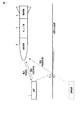•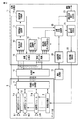•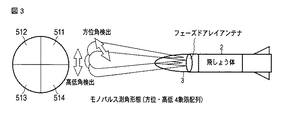•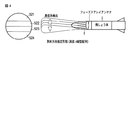•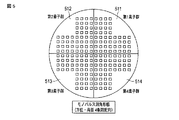•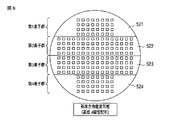•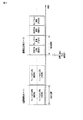•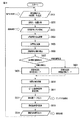•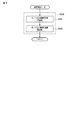•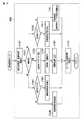•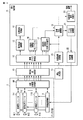•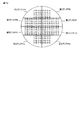•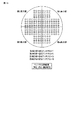•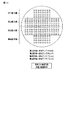•## Abstract

<P>PROBLEM TO BE SOLVED: To provide a guidance device capable of always detecting the direction of a target, and a target detection method. <P>SOLUTION: A switching type divider/combiner 9 divides an arrangement of a plurality of antenna elements 6-1 to 6-N into a plurality of regions in accordance with a mono-pulse angle measurement form or an arrival direction estimation form and combines reception power of a reflected wave received for each region. An error angle calculator 16 detects at least one of an azimuth angle and an angle of site of the target on the basis of the reception power of a region divided by the mono-pulse angle measurement form. An error angle calculator 17 separates a direct reflected wave and a ground reflected wave from the target on the basis of the reception power of the plurality of regions divided by the arrival direction estimation form, and detects the angle of site of the target. When the relative distance to the target is less than a prescribed distance, control is performed by an angle measurement form switch 14 so that the angle measurement by the mono-pulse angle measurement form and the angle measurement by the arrival direction estimation form are alternately performed. <P>COPYRIGHT: (C)2011,JPO&INPIT

## Description

The present invention relates to a guidance device for a flying object and a target detection method.

A flying object that tracks a target flying in the low sky by radio waves receives both a reflected wave reflected from the target by a guidance device mounted on the flying object and a reflected wave reflected from the ground surface and the sea surface. When the guidance device uses a normal monopulse angle measurement method, the reflected wave from the target and the reflected wave from the ground surface (or the sea surface) are combined and received. For this reason, depending on the synthesis situation, the detection result of the target direction varies greatly between the reflected wave from the target and the reflected wave from the ground surface (or the sea surface).

When a direction close to a reflected wave from the ground surface (or the sea surface) is detected as a target direction, the direction is a direction below the ground surface. Therefore, when the flying object has a low altitude, the flying object may collide with the ground surface. In order to prevent the flying object from colliding with the ground surface, it is necessary to detect separately the reflected wave from the target and the reflected wave from the ground surface (or the sea surface). For example, Non-Patent Document 1 describes a direction-of-arrival estimation method that determines the number of incoming waves when a plurality of waves arrive and estimates the direction of arrival of each wave.

Yamada, "Basics and Practice of High-Resolution Arrival Wave Estimation Methods", Design / Analysis Method Workshop for Antennas and Propagation (33rd), p.92, IEICE, 2006

However, the arrival direction estimation method described above is applied to a linear array antenna and cannot always detect the target azimuth and elevation angle. Moreover, the direction of arrival estimation method cannot be used unless the intensity of the received wave is large to some extent. For this reason, it is not possible to accurately track a target flying in a low sky.

The present invention has been made in view of the above problems, and an object thereof is to provide a guidance device and a target detection method that can always detect a target direction.

A guidance device according to an embodiment of the present invention transmits a radio wave to a target flying in the air, and the first reflected wave reflected by the target and the first reflected wave are caused by the ground surface or the sea surface. In accordance with transmission / reception means comprising a plurality of antenna elements for receiving at least one of the reflected second reflected waves, and a first form for monopulse angle measurement or a second form for direction of arrival estimation A power combining means for dividing the array of the plurality of antenna elements into a plurality of regions and combining the received power of the reflected waves received for each region, and at least one of the target azimuth and elevation angle The first angle detecting means for detecting one by monopulse angle measurement based on the received power of the plurality of areas divided in the first form, and the reception of the plurality of areas divided in the second form From power, said The first reflected wave and the second reflected wave are separated by direction-of-arrival estimation, and the relative angle between the second angle detection means for detecting the target elevation angle and the target is the total reception of the plurality of regions. When the relative distance calculated by the distance calculating means calculated based on the power and the distance calculating means is equal to or greater than a predetermined distance, the first angle detecting means detects the target azimuth and elevation angle, When the relative distance is less than a predetermined distance, detection of the target azimuth angle by the first angle detection unit and detection of the elevation angle of the target by the second angle detection unit are alternately performed. Detection control means for calculating, a direction calculation means for calculating the direction of the target based on the azimuth angle and elevation angle of the target detected by the first or second angle detection means, and the direction calculation means Based on the target direction Comprising a steering signal generating means for generating a steering signal spacecraft Te.

In addition, the target detection method according to an embodiment of the present invention transmits a radio wave to a target flying in the air with a plurality of antenna elements, the first reflected wave reflected by the target, and the first A transmission / reception step of receiving at least one of the second reflected waves reflected by the ground surface or the sea surface, and a first mode for monopulse angle measurement or a second mode for direction of arrival estimation Accordingly, the power combining step of dividing the array of the plurality of antenna elements into a plurality of regions and combining the received power of the reflected waves received for each region, and at least the target azimuth and elevation angle Either one of the first angle detecting step for detecting by monopulse angle measurement based on the received power of the plurality of regions divided in the first form, and the plurality of regions divided in the second form. The first reflected wave and the second reflected wave are separated from the received power by arrival direction estimation, and a relative angle between the target and a second angle detecting step for detecting the target elevation angle, If the distance calculation step is calculated based on the total received power of the plurality of regions, and the calculated relative distance is equal to or greater than a predetermined distance, the target azimuth and elevation angle by the first angle detection step When the relative distance is less than a predetermined distance, the detection of the azimuth angle of the target by the first angle detection step and the elevation angle of the target by the second angle detection step are performed. Direction calculation for calculating the direction of the target based on the detection control step for performing detection alternately and the azimuth angle and elevation angle of the target detected by the first or second angle detection step Comprising the step.

According to the present invention, when the relative distance to the target is less than the predetermined distance, the detection of the target elevation angle by the arrival direction estimation from the received power synthesized in the second form, and the first form Since the detection of the target azimuth by monopulse angle measurement from the combined received power is alternately performed, the target direction can always be detected.

The schematic diagram which shows an example of the target capture | acquisition by the flying body which mounts the guidance device which concerns on the 1st Embodiment of this invention. The block diagram which shows the detailed structure of the guidance device which concerns on 1st Embodiment. The figure which shows an example of the electric power synthetic | combination at the time of performing monopulse angle measurement. The figure which shows an example of the electric power synthetic | combination at the time of performing arrival direction estimation. The figure which shows the monopulse angle measurement form which concerns on 1st Embodiment. The figure which shows the arrival direction estimation form which concerns on 1st Embodiment. The schematic diagram which shows a mode that a measurement mode is switched according to the distance with a target in 1st Embodiment. The flowchart which shows the operation | movement procedure of a signal processing part. The flowchart which shows the angle measurement procedure in normal angle measurement mode. The flowchart which shows the angle measurement procedure in high precision angle measurement mode. The block diagram which shows the detailed structure of the guidance device which concerns on 2nd Embodiment. The figure which shows the unit of the electric power combination by the distribution combiner | synthesizer which concerns on 2nd Embodiment. The figure which shows the monopulse angle measuring form which concerns on 2nd Embodiment. The figure which shows the arrival direction estimation form which concerns on 2nd Embodiment The schematic diagram which shows a mode that a measurement mode is switched according to the distance with a target in 2nd Embodiment.

Hereinafter, embodiments of a guidance device according to the present invention will be described with reference to the drawings.

First Embodiment FIG. 1 is a schematic diagram showing an example of target capture by a flying object equipped with a guidance device according to a first embodiment of the present invention.

The flying body 2 includes a guidance device 3 and a steering device 4, and detects and follows the position of the target 1 flying in the air. The guidance device 3 outputs a guidance signal for the flying object 2 to fly in the direction of the target 1. The steering device 4 performs steering control to change the attitude of the flying object 2 in the direction of the target 1 in accordance with the guidance signal.

The guidance device 3 transmits radio waves (transmission waves) into the air and receives reflected radio waves from the target 1. From the received reflected wave, the distance between the target 1 and the flying object 2, the direction of the target 1, and the like are detected.

When the target 1 is flying, for example, in the low sky of about several tens of meters from the ground surface, the reflected radio wave from the target 1 may be further reflected on the ground or the sea surface (multipath). In such a case, the guidance device 3 receives both the reflected radio wave (directly reflected wave) reflected directly from the target 1 and the reflected radio wave (ground reflected wave) reflected from the ground or the like.

When the guidance device 3 receives the ground reflected wave, the position of the target virtual image (θ1 = θ2 in FIG. 1) that is symmetrical to the target 1 with respect to the ground surface or the sea surface, that is, below the ground surface or below the sea surface. The position may be erroneously detected as the target 1 position. If a position lower than the actual position of the target 1 such as below the ground surface is erroneously detected as the position of the target 1, the guidance signal output from the guidance device 3 also indicates a position lower than the actual position of the target 1. The steering device 4 steers the flying object 2 aiming at a lower altitude than the target 1. For this reason, the flying object 2 which flies at a speed of Mach unit may collide with the ground surface or the sea surface. In order to prevent such a collision, the guidance device 3 according to the present embodiment has a function of receiving a direct reflected wave and a ground reflected wave separately.

FIG. 2 is a block diagram illustrating a detailed configuration of the guidance device according to the first embodiment.

The guidance device 3 includes an antenna unit 5, a high frequency unit 10, a signal processing unit 11, and an inertia measurement unit 19.

The antenna unit 5 is formed by a phased array antenna. The antenna unit 5 includes a plurality of electronic scanning antenna elements 6-1 to 6-N, and includes transmission / reception modules 7-1 to 7-N corresponding to the respective antenna elements. The antenna elements 6-1 to 6-N are two-dimensionally arranged as shown in FIGS. 5 and 6, for example.

The antenna unit 5 also includes a phase shift amount controller 8 and a switching type distribution synthesizer 9. The phase shift amount controller 8 sets the phase shift amount in the transmission / reception modules 7-1 to 7-N. The transmission / reception modules 7-1 to 7-N form a beam in a predetermined direction based on the set phase shift amount, and transmit and receive radio waves via the antenna elements 6-1 to 6-N. The switching type distribution synthesizer 9 switches the form of combining received power of the transmission / reception modules 7-1 to 7-N based on a switching signal from the signal processing unit 11. Four systems of received signals corresponding to the switching are output to the high frequency unit 10.

The high frequency unit 10 converts each of the four received signals output from the switching type distribution synthesizer 9 from a radio frequency in GHz units to an intermediate frequency in MHz units and outputs the converted signals to the signal processing unit 11.

The inertial measurement unit 19 includes, for example, an angular velocity sensor and an acceleration sensor, and measures inertial measurement information (angular velocity and acceleration) of the flying object 2.

The signal output unit 11 includes a processor, a program memory, and a work memory (not shown). The processor executes a predetermined program stored in the program memory, thereby realizing the functions of the respective units as described below.

The signal processing unit 11 includes a power combining calculator 12, a target detection calculator 13, an angle measurement mode switch 14, a received data processor 15, error angle calculators 16 and 17, a target direction calculator 18, and a position / orientation calculator 20. , A beam command angle calculator 21, a phase shift amount calculator 22, and a steering command signal generator 23.

The position / orientation calculator 20 calculates the flight position and attitude angle of the flying object 2 based on the inertial measurement information (angular velocity and acceleration) of the flying object 2 measured by the inertia measuring unit 19.

The reception data processor 15 includes an A / D converter, and the A / D converter converts a reception signal input from the high frequency unit 10 into a digital signal.

The power combining calculator 12 combines the four systems of received power that have been digitally converted. The target detection calculator 13 determines whether or not the combined received power value is equal to or higher than a predetermined level. If the received power value does not reach a predetermined level, the received signal is assumed to be noise. On the other hand, if the received power value is equal to or higher than a predetermined level, it is considered that the reflected wave from the target 1 is being measured. If the received power value is equal to or higher than a predetermined level, the target detection calculator 13 calculates the relative distance from the flying object 2 to the target 1 based on the timing of the data samples. The angle measurement mode switch 14 instructs the switching distributor 9 and the reception data processor 15 to switch the angle measurement mode based on the information on the relative distance to the target 1.

The reception data processor 15 inputs from the high-frequency unit 11 to either the error angle calculator (monopulse) 16 or the error angle calculator (arrival direction estimation) 17 in response to an instruction from the angle measurement mode switch 14. Output four received signals.

The error angle calculator 16 performs a calculation according to monopulse angle measurement, and calculates an error angle in the azimuth direction (azimuth angle) and an error angle in the elevation direction (altitude angle).

On the other hand, the error angle calculator (direction-of-arrival estimation) 17 calculates an error angle in the height direction by a direction-of-arrival estimation method (MODE (method of direction estimation) method or MUSIC (multiple signal classification) method). When two reflected waves including a direct reflected wave and a ground reflected wave are received, the error angle calculator 17 calculates an error angle for each reflected wave. Based on the position and orientation angle of the flying object 2 obtained from the position / orientation computing unit 20, the error angle computing unit 17 estimates and selects the sky angle of the obtained error angles as the target 1 elevation angle. To do.

The target direction angle calculator 18 calculates the direction of the target 1 based on the azimuth angle and the elevation angle output from the error angle calculator 16 or the error angle calculator 17. The beam instruction angle calculator 21 calculates a new direction in which the beam should be directed, and the phase shift amount calculator 22 calculates phase shift amount data set in the antenna unit 5 and outputs it to the antenna unit 5.

The steering command signal generator 23 generates a guidance signal (steering command signal) for guiding the flying direction of the flying object 2 in the direction of the target 1 and outputs it to the steering device 4.

Next, the operation of the guidance device 3 configured as described above will be described.

FIG. 3 is a diagram illustrating an example of power combining when performing monopulse angle measurement.

The monopulse angle measurement is used to measure the elevation angle and azimuth angle of the target 1. At the time of monopulse angle measurement, the arrangement of the antenna elements 6-1 to 6-N of the antenna unit 5 is equally divided into four parts (four quadrant arrangement). As shown in FIG. 5, the antenna elements included in the upper right region 511 are the first element group, the antenna elements included in the upper left region 512 are the second element group, and the antenna elements included in the lower left region 513 are the third element. The antenna elements included in the group, lower right region 514, are defined as a fourth element group. The switchable distribution synthesizer 9 synthesizes received power for each element group and generates four systems of received signals. The error angle calculator 16 detects the azimuth angle and elevation angle of the target 1 from this received signal.

FIG. 4 is a diagram illustrating an example of power combining when direction of arrival estimation is performed.

The direction of arrival estimation is used to separate the direct reflected wave and the ground reflected wave and estimate the elevation angle of the target 1. At the time of arrival direction estimation, the array of antenna elements 6-1 to 6-N of the antenna unit 5 is divided into four regions 521 to 524 in the vertical direction (four vertical arrays). As shown in FIG. 6, the antenna elements included in the uppermost region 521 are included in the first element group, the antenna elements included in the second region 522 from the top are included in the second element group, and the third region 513 from the top. An antenna element included in the lowermost region 514 is referred to as a fourth element group. The switchable distribution synthesizer 9 synthesizes received power for each element group and generates four systems of received signals. The error angle calculator 17 detects the target reflected wave and the ground reflected wave from the received signal by the arrival direction estimation process (MUSIC method or MODE method), and detects the elevation angle of the target 1 from the upper reflected wave. By directing the arrival direction, the direct reflected wave and the ground reflected wave can be detected separately.

FIG. 7 is a schematic diagram showing how the measurement mode is switched according to the distance from the target in the first embodiment.

The flying body 2 is configured as a small device having a size of several tens of centimeters or less, and a phased array antenna type antenna unit 5 is mounted on the front portion. When the target 1 flying in the low sky is far away and the relative distance to the flying object 2 is large, the error angle is detected in the normal angle measurement mode. In the normal angle measurement mode, the elevation angle and the azimuth angle are detected from the received signal received in one detection period by monopulse angle measurement (see FIGS. 3 and 5).

When the flying object 2 approaches the target 1 that flies in the low sky (for example, the distance to the target 1 is about ½ of the distance first detected) and the flying altitude of the flying object 2 itself decreases (for example, several tens of times) M), the error angle is detected in the high-precision angle measurement mode. In the high-accuracy angle measurement mode, one detection period is divided into front and rear, power combining is performed according to the direction of arrival estimation mode (see FIGS. 4 and 6), and the error angle calculator 17 detects the height angle, then the monopulse measurement. The power is synthesized by the angle (see FIGS. 3 and 5), and the error angle calculator 16 detects the azimuth angle. Although the influence of the ground reflected wave is remarkably generated in the detection of the elevation angle of the target 1, the antenna elements 6-1 to 6-N are allocated to the regions 521 to 524 in tandem and the direction of arrival estimation process is performed. The elevation angle can be detected by separating the reflected wave and the ground reflected wave. On the other hand, the detection of the azimuth angle of the target 1 has almost no influence of the ground reflected wave. Therefore, the azimuth angle is detected by monopulse angle measurement.

As described above, in the guidance device 3 according to the present embodiment, when the flying object 2 approaches the target 1 that flies in the low sky, the power combining mode of the phased array antenna is switched to change the elevation angle (direction of arrival estimation) and azimuth angle. (Monopulse) is detected alternately. Thereby, it is possible to continue high-precision detection with respect to a target flying in the low sky.

Next, the operation procedure of the signal processing unit 11 will be described.

FIG. 8 is a flowchart showing an operation procedure of the signal processing unit 11.

First, inertia information (angular velocity, acceleration) measured by the inertia measuring unit 19 is input to the position / orientation calculator 20 (step S801), and the position / orientation calculator 20 calculates the position and the position of the flying object 2 based on the inertia information. An attitude angle is calculated (step S802).

The four received signals synthesized in the monopulse angle measurement form or the arrival direction estimation form in accordance with the setting of the switching type distribution synthesizer 9 are input to the power composition calculator 12 via the reception data processing unit 15 (step S803). The power combining calculator 12 adds the four received signals, and calculates the total power of the received signals (step S805). When the total power of the received signal is equal to or greater than a predetermined value, it is determined that a reflected wave from the target 1 has been detected. When the reflected wave from the target 1 is detected, the target detection calculator 13 calculates the relative distance from the target 1 based on the time required from sending the transmission wave to receiving the reflected wave. When the total power of the received signal is less than the predetermined value, the processing from steps S801 to S804 may be repeated by returning to step S801.

The angle measuring form switching unit 14 determines whether or not the calculated relative distance to the target 1 is equal to or greater than a predetermined distance (step S807). If the relative distance is equal to or greater than the predetermined distance, the angle measurement mode switching unit 14 instructs the switching distribution synthesizer 9 and the reception data processor 15 to perform angle measurement in the normal angle measurement mode (step S808). On the other hand, if the relative distance is less than the predetermined distance, the angle measurement mode switching unit 14 instructs the switching type distributor 9 and the reception data processor 15 to perform angle measurement in the high accuracy measurement mode (step S809).

FIG. 9 is a flowchart showing the angle measurement procedure in the normal angle measurement mode.

When the normal angle measurement mode is designated, the reception data processor 15 outputs four received signals in the monopulse angle measurement mode (see FIGS. 3 and 5) to the error angle calculator 16. The error angle calculator 16 includes the sum of received power of the second element group (upper left area 512) and the third element group (lower left area 513), the first element group (upper right area 511), and the fourth element group. A difference from the sum of received power in (lower right region 514) is calculated, and an azimuth angle of target 1 is calculated from the difference (step S901). Further, the error angle calculator 16 includes the sum of received power of the first element group (upper right area 511) and the second element group (upper left area 512), the third element group (lower left area 513), and the fourth. A difference with the sum of received power of the element group (lower right region 514) is calculated, and an elevation angle of the target 1 is calculated from the difference (step S902).

In FIG. 9, the measurement of the elevation angle (step S902) is performed after the measurement of the azimuth angle of the target 1 (step S901). Conversely, the azimuth angle is measured after the elevation angle of the target 1 is measured. May be. When the azimuth angle and elevation angle of target 1 are measured, the flow proceeds to step S810 in FIG.

FIG. 10 is a flowchart showing the angle measurement procedure in the high-precision angle measurement mode.

When the high-accuracy angle measurement mode is designated, the reception data processor 15 outputs four received signals in the arrival direction estimation form (see FIGS. 4 and 6) to the error angle calculator 17. The error angle calculator 17 performs the direction of arrival estimation calculation by the MUSIC method or the MODE method, and calculates the error angle in the height direction (step S1001).

The error angle calculator 17 determines the number of detected radio waves (the number of received reflected waves) (step S1002). If two radio waves are received, it is determined that both the target reflected wave and the ground reflected wave are detected, and the error angle calculator 17 sets the two-wave determination flag to “ON” in the work memory (step S1). S1003). The error angle calculator 17 acquires the position and posture angle of the flying object 2 calculated by the position / orientation calculator 20 (step S1004). Based on this position and attitude angle and the beam indication angle obtained in the previous detection period, the radio wave indicating the upper side of the two waves is set as the target reflected wave, and the error angle calculator 17 calculates the error angle of the target reflected wave. Is selected as the elevation angle of the target 1 (step S1005).

When one radio wave is received, it is determined that two waves have not been detected due to the influence of power fluctuation or the like, and the error angle calculator 17 determines the setting of the two-wave determination flag (step S1006). If the two-wave determination flag is set to “On”, two-wave detection has already been performed, and the elevation angle value obtained by determining the upper target in the previous two-wave detection is used. A prediction filter (αβ filter, Kalman filter, etc.) is applied to the estimation value of the elevation angle (step S1007). If the two-wave determination flag is set to “OFF”, two-wave detection has not yet been performed, so the error angle calculator 17 selects the error angle calculated in step S1001 as the target 1 elevation angle. (Step S1008).

If no radio wave has been received, it is similarly determined that two waves have not been detected due to the influence of power fluctuation or the like, and the error angle calculator 17 determines the setting of the two-wave determination flag ( Step S1009). When the setting of the two-wave determination flag is “ON”, a prediction filter (αβ filter, Kalman filter, etc.) is applied to the elevation angle obtained in the previous angle measurement process to estimate the elevation angle prediction value ( Step S1010). When the setting of the two-wave determination flag is “OFF”, the error angle calculator 17 determines from the angle measurement processing results up to the previous time including the detection result of the elevation angle by the monopulse angle measurement mode in the normal angle measurement mode. A prediction filter is applied to predict the elevation angle, and is selected as the elevation angle of target 1 (step S1011).

When the elevation angle of the target 1 is selected, the angle measurement form switch 14 switches the angle measurement form to the monopulse angle measurement form (see FIGS. 3 and 5). The error angle calculator 16 calculates the azimuth angle of the target 1 from the four received signals in the monopulse angle measurement mode (step S1012). When the azimuth angle and elevation angle of the target 1 are measured, the flow proceeds to step S810 in FIG.

When the azimuth angle and elevation angle of the target 1 are calculated by the processing of step S808 or step S809, the target direction angle calculator 18 obtains the azimuth angle and elevation angle, the attitude angle of the flying object 2, and the previous detection period. The direction of target 1 (target direction angle) is calculated based on the obtained beam instruction angle (step S810). Then, the beam pointing angle calculator 21 calculates a beam pointing angle at which a beam should be newly directed based on the target direction angle, and phase shift amount data necessary for emitting the transmission wave beam to the beam pointing angle is phase-shifted. The quantity calculator 22 calculates (step S811). The calculated phase shift amount data is output to the antenna unit 5 (step S812).

The steering command signal generator 23 generates a steering command signal based on the error angle (step S813) and outputs the steering command signal to the steering device 4 (step S814). Thereafter, the flow returns to step S801, and the same measurement is repeated.

As described above, according to the guidance device 3 according to the present embodiment, when the flying object 2 approaches the target 1, only the direct reflected wave can be captured and tracked.

The antenna unit 5 uses a phased array antenna system based on phase control, and performs power measurement of the antenna elements 6-1 to 6-N so that both normal angle measurement (monopulse angle measurement) and angle measurement based on arrival direction estimation can be performed. A switching type combiner / distributor 9 capable of switching the combining form is provided. The power combining mode by the switching type combiner / distributor 9 can be switched according to the control signal from the signal processing unit 11. The signal processing unit 11 includes a signal processing unit (error angle calculator 16) that performs monopulse angle measurement and a signal processing unit (error angle calculator 17) that performs arrival direction estimation in order to detect the azimuth angle and elevation angle of the target 1. ), A series of functions can be realized even in the small flying object 2.

In the guidance device 3, when the target 1 and the flying object 2 approach each other, it is possible to detect the direct reflected wave and the ground reflected wave separately by applying the arrival direction estimation process to the angle detection in the elevation direction. Further, after detecting the direct reflected wave and the ground reflected wave separately, the vertical direction is estimated based on the inertia information. Thereby, the direction of the direct reflected wave is estimated, and the direction of the target 1 can be estimated.

Further, according to the present embodiment, the direction of the target 1 is estimated based on the already detected information even if the direct reflected wave and the target reflected wave cannot be separated and detected due to radio wave environment factors such as power fluctuations. Therefore, tracking can be continued.

Next, another embodiment of the present invention will be described. In the following embodiments, portions corresponding to those of the first embodiment are denoted by corresponding reference numerals, and detailed description thereof is omitted.

In the first embodiment, the flying object 2 is small and the number of reception systems is limited to four. However, when there is a sufficient mounting scale, the number of reception systems is eight as in the second embodiment. It is good.

Second Embodiment FIG. 1 also shows an example of target capture by a flying object equipped with a guidance device according to a second embodiment.

FIG. 11 is a block diagram illustrating a detailed configuration of the guidance device according to the second embodiment.

The guidance device 3 includes an antenna unit 5, a high frequency unit 10, a signal processing unit 11, and an inertial measurement unit 19, as in the first embodiment.

Similarly to the first embodiment, the antenna unit 5 is formed by a phased array antenna, and includes a plurality of electronic scanning antenna elements 6-1 to 6-N and transmission / reception modules 7-1 to 7-1 corresponding to the respective antenna elements. 7-N. The antenna elements 6-1 to 6-N are two-dimensionally arranged as shown in FIGS.

The antenna unit 5 also includes a phase shift amount controller 8 and a distribution synthesizer 90. The distribution synthesizer 90 according to the present embodiment synthesizes the reception power of the transmission / reception modules 7-1 to 7-N to generate eight systems of reception signals.

FIG. 12 shows a unit of power combining by the distribution combiner 90 according to the second embodiment. As shown in FIG. 12, the arrangement of the antenna elements 6-1 to 6-N of the antenna unit 5 is equally divided into left and right, and the left and right regions are each divided into four regions vertically. That is, the array of antenna elements 6-1 to 6-N is divided into a total of eight regions. Each of the divided areas constitutes eight partial antennas A to H. The distribution synthesizer 90 sums the received power of the antenna elements included in each partial antenna, generates eight systems of received signals, and outputs them to the high frequency unit 10.

The high frequency unit 10 converts each of the eight received signals output from the distribution synthesizer 90 from a radio frequency in GHz units to an intermediate frequency in MHz units and outputs the converted signals to the signal processing unit 11.

The inertial measurement unit 19 includes, for example, an angular velocity sensor and an acceleration sensor, and measures inertial measurement information (angular velocity and acceleration) of the flying object 2.

The signal processing unit 11 according to the second embodiment includes a power combining calculator 12, a target detection calculator 13, a received data processor 15, error angle calculators 16 and 17, a target direction calculator 18, and a position / orientation calculator 20. , A beam command angle calculator 21, a phase shift amount calculator 22, and a steering command signal generator 23.

The power combining calculator 12 combines the eight systems of received power that have been digitally converted. The target detection calculator 13 determines whether or not the combined received power value is equal to or higher than a predetermined level. If the received power value does not reach a predetermined level, the received signal is assumed to be noise. On the other hand, if the received power value is equal to or higher than a predetermined level, it is considered that the reflected wave from the target 1 is being measured. If the received power value is greater than or equal to a predetermined level, the target detection calculator 13 calculates the relative distance from the flying object 2 to the target 1.

The reception data processor 15 generates four systems of signals based on the eight systems of received signals input from the high-frequency unit 11, and an error angle calculator (monopulse) 16 and an error angle calculator (arrival direction estimation) 17. Output to.

The error angle calculator 16 performs a calculation according to monopulse angle measurement, and calculates an error angle in the azimuth direction (azimuth angle) and an error angle in the elevation direction (altitude angle).

The monopulse angle measurement is used to measure the elevation angle and azimuth angle of the target 1 as in the first embodiment. During monopulse angle measurement, the arrangement of the antenna elements 6-1 to 6-N of the antenna unit 5 is equally divided (up to four quadrants) vertically and horizontally as in the first embodiment.

That is, as shown in FIG. 13, the antenna elements of the partial antenna E and the partial antenna F represent the first element group on the upper right, the antenna elements of the partial antenna A and the partial antenna B represent the second element group on the upper left, and the partial antenna C. In addition, the antenna elements of the partial antenna D constitute the lower left third element group, and the antenna elements of the partial antenna G and the partial antenna H constitute the lower right fourth element group. The distribution synthesizer 9 synthesizes the received power for each element group and generates four systems of received signals. The error angle calculator 16 detects the azimuth angle and elevation angle of the target 1 from this received signal.

The error angle calculator (arrival direction estimation) 17 calculates an error angle in the height direction by the arrival direction estimation method (MODE method or MUSIC method).

Similar to the first embodiment, the arrival direction estimation is used to separate the direct reflected wave and the ground reflected wave and estimate the elevation angle of the target 1. At the time of arrival direction estimation, the antenna elements 6-1 to 6-N of the antenna unit 5 are divided into four regions in the vertical direction (four vertical arrays), as in the first embodiment.

That is, as shown in FIG. 14, the antenna elements of partial antenna A and partial antenna E are the uppermost first element group, and the antenna elements of partial antenna B and partial antenna F are the second second element group from the top. The antenna elements of the partial antenna C and the partial antenna G constitute the third third element group from the top, and the antenna elements of the partial antenna D and the partial antenna H constitute the lowermost fourth element group. Distribution synthesizer 90 synthesizes received power for each element group and generates four systems of received signals. The error angle calculator 17 detects the target reflected wave and the ground reflected wave from the received signal by the arrival direction estimation process (MUSIC method or MODE method), and detects the elevation angle of the target 1 from the upper reflected wave. The target reflected wave and the ground reflected wave can be separated and detected by the arrival direction estimation.

The target direction angle calculator 18 calculates the direction of the target 1 based on the azimuth angle and the elevation angle output from the error angle calculator 16 and the error angle calculator 17. The beam instruction angle calculator 21 calculates a new direction in which the beam should be directed, and the phase shift amount calculator 22 calculates phase shift amount data set in the antenna unit 5 and outputs it to the antenna unit 5.

The steering command signal generator 23 generates a guidance signal (steering command signal) for guiding the flying direction of the flying object 2 in the direction of the target 1 and outputs it to the steering device 4.

FIG. 15 is a schematic diagram showing how the measurement mode is switched according to the distance from the target in the second embodiment.

When the target 1 flying in the low sky is far away and the relative distance to the flying object 2 is large, the error angle is detected in the normal angle measurement mode. In the normal angle measurement mode, as in the first embodiment, the elevation angle and azimuth angle are detected by monopulse angle measurement from the received signal received in one detection period.

When the flying object 2 approaches the target 1 that flies in the low sky and the flying altitude of the flying object 2 decreases, the error angle is detected by the high-precision angle measurement mode. In the high-precision angle measurement mode according to the second embodiment, it is possible to detect both the elevation angle according to the arrival direction estimation form and the azimuth angle according to the monopulse angle measurement from the received signal received during one detection period.

As described above, the guidance device according to the present embodiment performs monopulse angle measurement (4-quadrant array) and direction-of-arrival estimation (4-vertical array) simply by calculating eight received signals input to the signal processing unit 11. be able to. Therefore, it is not necessary to configure the combiner / distributor in a switching type. Further, simultaneous processing of monopulse angle measurement and direction-of-arrival estimation can be performed by a received signal in one detection period.

The present invention is not limited to the above-described embodiments, and various modifications can be made without departing from the scope of the invention when it is practiced. Further, each of the embodiments includes inventions at various stages, and various inventions can be extracted by appropriately combining a plurality of disclosed constituent elements. For example, even if some constituent elements are deleted from all the constituent elements shown in one embodiment or the constituent elements shown in some embodiments are combined, they are described in the column of the problem to be solved by the invention. In the case where the problems described above can be solved and the effects described in the “Effects of the Invention” can be obtained, a configuration in which these constituent requirements are deleted or combined can be extracted as an invention.

DESCRIPTION OF SYMBOLS 1 ... Target, 2 ... Flying object, 3 ... Guidance device, 4 ... Steering device, 5 ... Antenna part, 6-1 to 6-N ... Antenna element, 7-1 to 7-N ... Transmission / reception module, 8 ... Transfer Phase amount controller, 9 ... switching type distribution synthesizer, 10 ... high frequency unit, 11 ... signal processing unit, 12 ... power synthesis computing unit, 13 ... target detection computing unit, 14 ... angle measurement mode switching unit, 15 ... received data Processor, 16, 17 ... Error angle calculator, 18 ... Target direction calculator, 19 ... Inertia measurement unit, 20 ... Position and orientation calculator, 21 ... Beam indication angle calculator, 22 ... Phase shift amount calculator, 23 ... Steering command signal generator.

## Claims (9)

1. A radio wave is transmitted to a target flying in the air, and at least one of a first reflected wave reflected by the target and a second reflected wave reflected by the ground surface or the sea surface. Transmitting and receiving means comprising a plurality of antenna elements for receiving one;
According to the first form for monopulse angle measurement or the second form for direction-of-arrival estimation, the array of the plurality of antenna elements is divided into a plurality of regions, and the received reflected wave is received for each region. Power combining means for combining power; and
First angle detection means for detecting at least one of the target azimuth angle and elevation angle by monopulse angle measurement based on the received power of a plurality of areas divided in the first form;
A second angle for detecting the target elevation angle by separating the first reflected wave and the second reflected wave from the received power of the plurality of regions divided in the second form by estimating the arrival direction. Detection means;
Distance calculating means for calculating a relative distance to the target based on a total received power of the plurality of regions;
When the relative distance calculated by the distance calculation unit is equal to or greater than a predetermined distance, the first angle detection unit detects the target azimuth angle and elevation angle, and the relative distance is less than the predetermined distance. Detection control means for alternately detecting the azimuth angle of the target by the first angle detection means and detecting the elevation angle of the target by the second angle detection means;
Direction calculating means for calculating the direction of the target based on the azimuth angle and elevation angle of the target detected by the first or second angle detecting means;
Steering signal generating means for generating a flying object steering signal based on the target direction calculated by the direction calculating means;
A guidance device comprising:
2. In the first form, the arrangement of the plurality of antenna elements is divided into four equal parts vertically and horizontally,
2. The induction device according to claim 1, wherein in the second embodiment, the arrangement of the plurality of antenna elements is divided into four regions in a column.
3.   When the relative distance is less than a predetermined distance, the detection control means switches the power combining form by the power combining means between the first form and the second form, and the first angle detecting means 2. The guidance device according to claim 1, wherein the detection of the azimuth angle of the target by means of and the detection of the elevation angle of the target by the second angle detection means are alternately performed.
4.   When either one of the first reflected wave and the second reflected wave is received by the transmitting / receiving means, the second angle detecting means detects the target detected by the direction of arrival estimation in the previous detection. The guidance device according to claim 1, wherein a predicted value of an elevation angle is estimated from an elevation angle.
5.   When the first reflected wave and the second reflected wave are not received by the transmission / reception means, the second angle detection means determines the elevation angle detected by monopulse angle measurement of the first angle detection means. The guidance device according to claim 1, wherein the guidance device is set as a target elevation angle.
6.   When the first reflected wave and the second reflected wave are received by the transmission / reception means, the second angle detection means is configured to detect an upper side of the first reflected wave and the second reflected wave. The guidance device according to claim 1, wherein a reflected wave is determined based on inertia information of the flying object, and the target elevation angle is detected from the upper reflected wave.
7. The flying object comprises a measuring means for measuring inertial information including the attitude angle and position of the flying object,
The guidance device according to claim 6, wherein the second angle detection unit determines a vertical direction based on the inertia information.
8. The arrangement of the plurality of antenna elements is divided into eight regions,
The induction device according to claim 1, wherein the power combining unit combines received power in each of the eight regions and calculates a combined result according to the first form or the second form.
9. A plurality of antenna elements transmit radio waves to a target flying in the air. The first reflected wave reflected by the target and the second reflected wave reflected by the ground surface or the sea surface A transmission / reception step of receiving at least one of the reflected waves;
According to the first form for monopulse angle measurement or the second form for direction-of-arrival estimation, the array of the plurality of antenna elements is divided into a plurality of regions, and the received reflected wave is received for each region. A power combining step of combining power;
A first angle detection step of detecting at least one of the target azimuth angle and elevation angle by monopulse angle measurement based on the received power of a plurality of regions divided in the first form;
A second angle for detecting the target elevation angle by separating the first reflected wave and the second reflected wave from the received power of the plurality of regions divided in the second form by arrival direction estimation. A detection step;
A distance calculating step of calculating a relative distance to the target based on a total received power of the plurality of regions;
When the calculated relative distance is equal to or larger than a predetermined distance, the target azimuth and elevation angle are detected by the first angle detection step, and the relative distance is less than the predetermined distance. A detection control step for alternately detecting the azimuth angle of the target by the first angle detection step and detecting the elevation angle of the target by the second angle detection step;
A direction calculating step of calculating the direction of the target based on the azimuth angle and elevation angle of the target detected by the first or second angle detecting step;
A target detection method comprising:
JP2009286677A 2009-12-17 2009-12-17 Guiding device and target detection method Active JP5380267B2 (en)

## Priority Applications (1)

Application Number Priority Date Filing Date Title
JP2009286677A JP5380267B2 (en) 2009-12-17 2009-12-17 Guiding device and target detection method

## Applications Claiming Priority (1)

Application Number Priority Date Filing Date Title
JP2009286677A JP5380267B2 (en) 2009-12-17 2009-12-17 Guiding device and target detection method

## Publications (2)

Publication Number Publication Date
JP2011128011A true JP2011128011A (en) 2011-06-30
JP5380267B2 JP5380267B2 (en) 2014-01-08

# Family

## Family Applications (1)

Application Number Title Priority Date Filing Date
JP2009286677A Active JP5380267B2 (en) 2009-12-17 2009-12-17 Guiding device and target detection method

## Country Status (1)

JP (1) JP5380267B2 (en)

## Cited By (3)

* Cited by examiner, † Cited by third party
Publication number Priority date Publication date Assignee Title
JP2013061214A (en) * 2011-09-13 2013-04-04 Toshiba Corp Target follow-up device, guidance device, and target follow-up method
JP2015025744A (en) * 2013-07-26 2015-02-05 日本電気株式会社 Radar device and flying target elevation calculation method
EP3276373A1 (en) * 2016-07-26 2018-01-31 Alpha Networks Inc. Mobile navigation method and system

## Citations (4)

* Cited by examiner, † Cited by third party
Publication number Priority date Publication date Assignee Title
JPH11194167A (en) * 1998-01-05 1999-07-21 Toshiba Corp Radar equipment
JP2001264427A (en) * 2000-03-22 2001-09-26 Toshiba Corp Radar
JP2002310597A (en) * 2001-04-04 2002-10-23 Mitsubishi Electric Corp Guided projectile and radar
JP2008098999A (en) * 2006-10-12 2008-04-24 Toshiba Corp Radar apparatus

## Patent Citations (4)

* Cited by examiner, † Cited by third party
Publication number Priority date Publication date Assignee Title
JPH11194167A (en) * 1998-01-05 1999-07-21 Toshiba Corp Radar equipment
JP2001264427A (en) * 2000-03-22 2001-09-26 Toshiba Corp Radar
JP2002310597A (en) * 2001-04-04 2002-10-23 Mitsubishi Electric Corp Guided projectile and radar
JP2008098999A (en) * 2006-10-12 2008-04-24 Toshiba Corp Radar apparatus

## Cited By (4)

* Cited by examiner, † Cited by third party
Publication number Priority date Publication date Assignee Title
JP2013061214A (en) * 2011-09-13 2013-04-04 Toshiba Corp Target follow-up device, guidance device, and target follow-up method
JP2015025744A (en) * 2013-07-26 2015-02-05 日本電気株式会社 Radar device and flying target elevation calculation method
EP3276373A1 (en) * 2016-07-26 2018-01-31 Alpha Networks Inc. Mobile navigation method and system
US10444343B2 (en) 2016-07-26 2019-10-15 Alpha Networks Inc. Mobile navigation method and system

## Also Published As

Publication number Publication date
JP5380267B2 (en) 2014-01-08

## Similar Documents

Publication Publication Date Title
Bellettini et al. Theoretical accuracy of synthetic aperture sonar micronavigation using a displaced phase-center antenna
US7250902B2 (en) Method of generating accurate estimates of azimuth and elevation angles of a target for a phased—phased array rotating radar
Chen et al. Simulation of ISAR imaging of moving targets
CN101765785B (en) A digital beam-forming apparatus and technique for a multi-beam global positioning system (GPS) receiver
US3833904A (en) Airborne switched array radar system
US4825213A (en) Simultaneous triple aperture radar
EP1118870A2 (en) Low cost angle of arrival measurement system
Dogancay UAV path planning for passive emitter localization
US8624773B2 (en) Multidirectional target detecting system and method
JP2008501953A (en) Method and system for improving the accuracy of a terrain-assisted navigation system
WO2010071502A1 (en) Measuring of a landing platform of a ship
WO2003067278A2 (en) System and method for doppler track correlation for debris tracking
WO2005116682A1 (en) An arrangement for accurate location of objects
US20070052580A1 (en) Method and apparatus for improved determination of range and angle of arrival utilizing a two tone cw radar
EP1954920B1 (en) System for identifying high-quality phase angle measurements in an interferometric radar system
DE10258367A1 (en) Multi-objective method and multi-objective sensor device for the distance and angle localization of target objects in the vicinity
WO2008053685A1 (en) Radar target detecting method and radar device using the target detection method
JP5009812B2 (en) Wireless-based positioning system using synthetic aperture
US6933888B1 (en) Multi-ship coherent geolocation system
US20160139254A1 (en) Short-Range Point Defense Radar
Gu et al. Optimal cooperative sensing using a team of UAVs
US8229472B2 (en) System and method for enabling determination of a position of a transponder
EP2420856B1 (en) Device and method of three-dimensional positioning
US5579011A (en) Simultaneous triple aperture radar

## Legal Events

Date Code Title Description
A621 Written request for application examination

Free format text: JAPANESE INTERMEDIATE CODE: A621

Effective date: 20120614

A977 Report on retrieval

Free format text: JAPANESE INTERMEDIATE CODE: A971007

Effective date: 20130828

TRDD Decision of grant or rejection written
A01 Written decision to grant a patent or to grant a registration (utility model)

Free format text: JAPANESE INTERMEDIATE CODE: A01

Effective date: 20130903

A61 First payment of annual fees (during grant procedure)

Free format text: JAPANESE INTERMEDIATE CODE: A61

Effective date: 20130930

R151 Written notification of patent or utility model registration

Ref document number: 5380267

Country of ref document: JP

Free format text: JAPANESE INTERMEDIATE CODE: R151